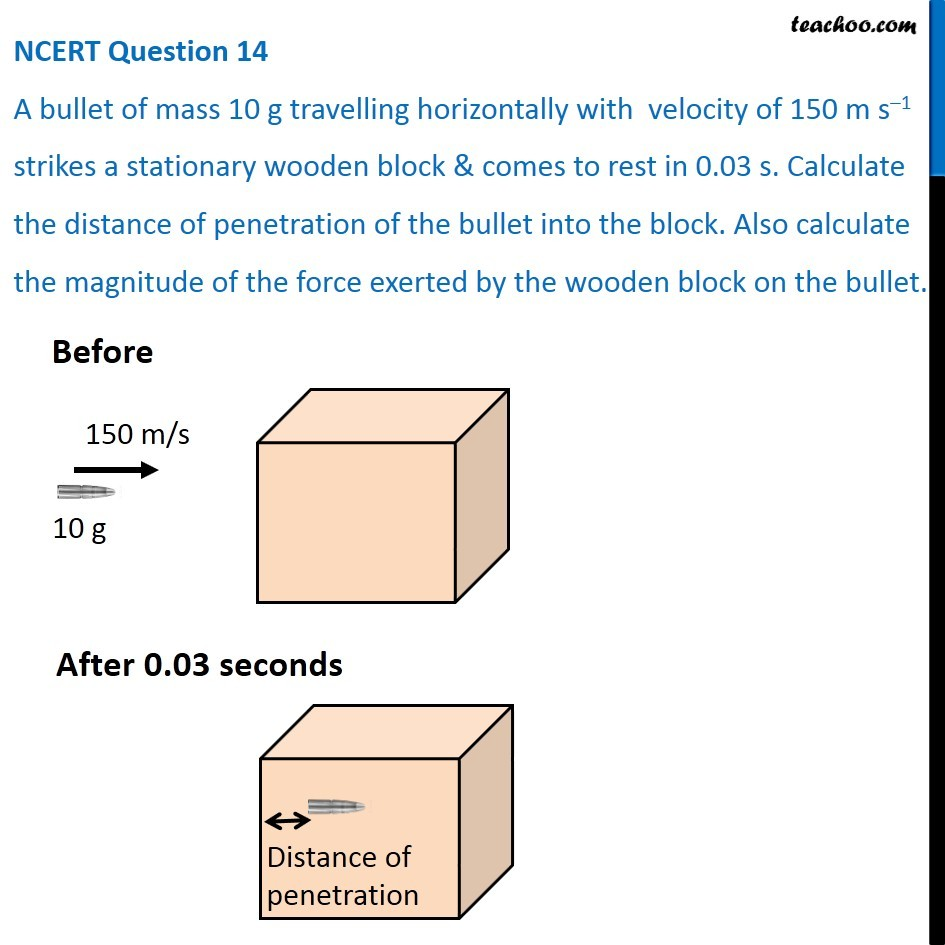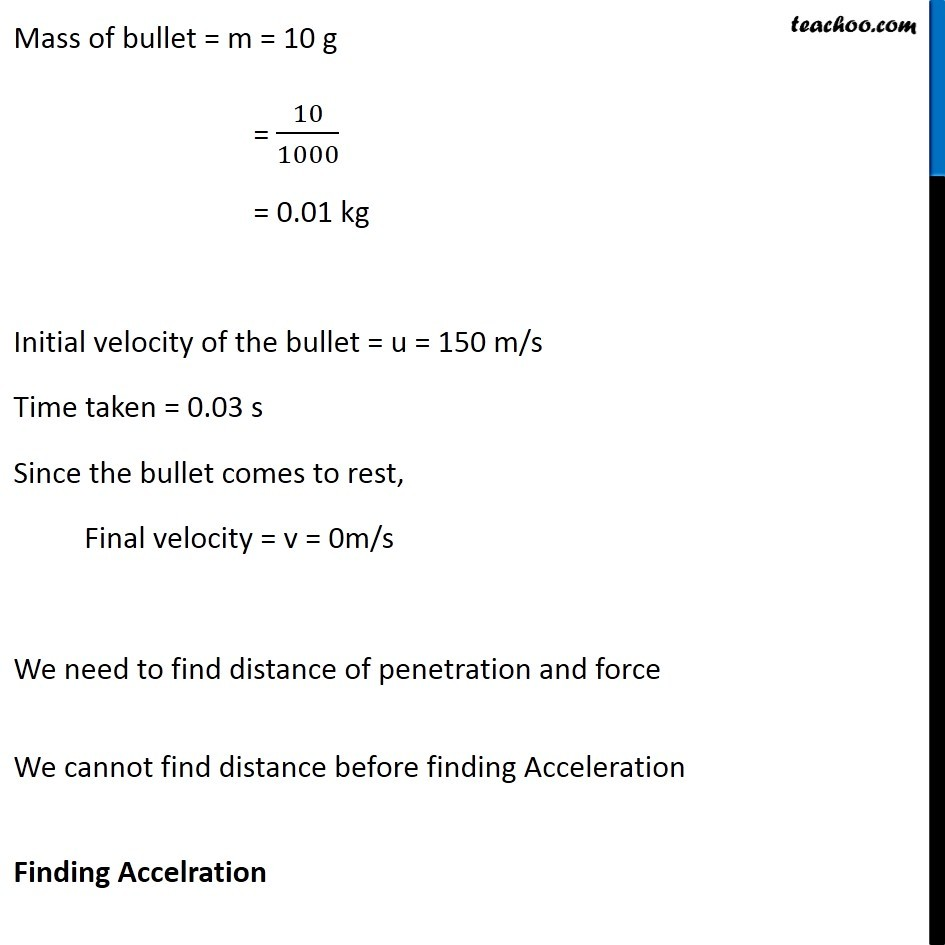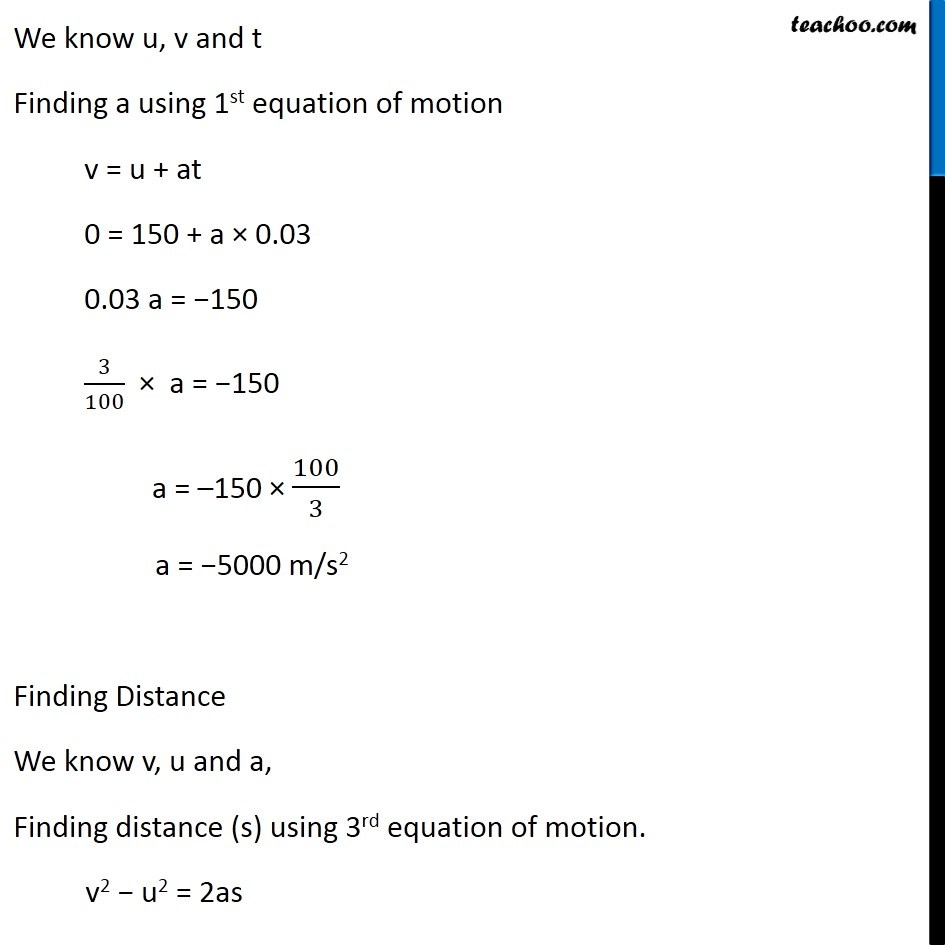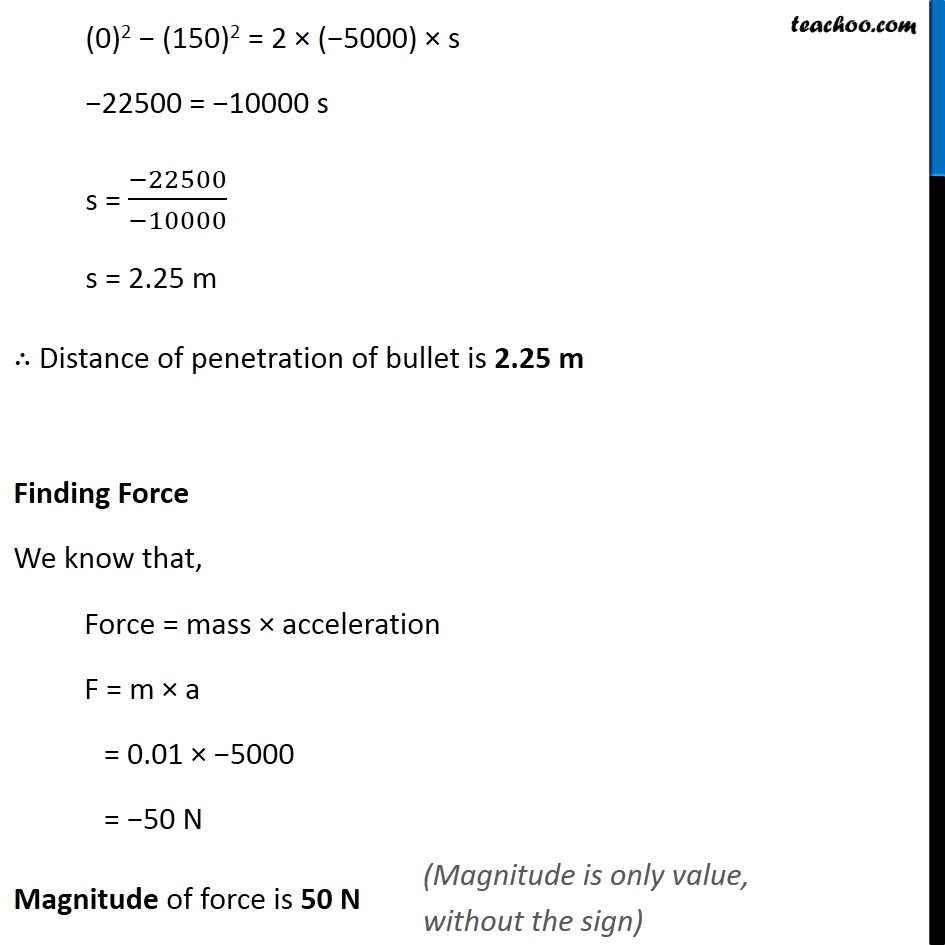NCERT Questions

Class 9
Chapter 9 Class 9 - Force and Laws Of MotionLearn in your speed, with individual attention - Teachoo Maths 1-on-1 Class

### Transcript

NCERT Question 14 A bullet of mass 10 g travelling horizontally with velocity of 150 m s–1 strikes a stationary wooden block & comes to rest in 0.03 s. Calculate the distance of penetration of the bullet into the block. Also calculate the magnitude of the force exerted by the wooden block on the bullet. Before 150 m/s After 0.03 seconds Distance of penetration Mass of bullet = m = 10 g = 10/1000 = 0.01 kg Initial velocity of the bullet = u = 150 m/s Time taken = 0.03 s Since the bullet comes to rest, Final velocity = v = 0m/s We need to find distance of penetration and force We cannot find distance before finding Acceleration Finding Accelration We know u, v and t Finding a using 1st equation of motion v = u + at 0 = 150 + a × 0.03 0.03 a = −150 3/100 × a = −150 a = –150 × 100/3 a = −5000 m/s2 Finding Distance We know v, u and a, Finding distance (s) using 3rd equation of motion. v2 − u2 = 2as (0)2 − (150)2 = 2 × (−5000) × s −22500 = −10000 s s = (−22500)/(−10000) s = 2.25 m ∴ Distance of penetration of bullet is 2.25 m Finding Force We know that, Force = mass × acceleration F = m × a = 0.01 × −5000 = −50 N Magnitude of force is 50 N (Magnitude is only value, without the sign)